## Thursday, March 8, 2018

### Simulating migration, natural selection, & genetic drift in R

I just added another function to my evolutionary genetics teaching R package, PopGen to simulate the simultaneous actions of migration, natural selection, & genetic drift.

Here is what the function code looks like:

``````msd<-function(p0=c(0.5,0.5),Ne=c(100,100),
w=list(c(1,1,1),c(1,1,1)),m=c(0.01,0.01),ngen=400,
colors=c("red","blue"),...){
if(hasArg(show.legend)) show.legend=list(...)\$show.legend
else show.legend<-TRUE
w<-lapply(w,function(w) (w/max(w))[3:1])
gametes<-lapply(Ne,function(Ne) rep(0,2*Ne))
gametes<-mapply(function(p0,g,N){
g[1:round(p0*2*N)]<-1
g},p0=p0,g=gametes,N=Ne,SIMPLIFY=FALSE)
p<-lapply(gametes,mean)
for(i in 1:ngen){
genotypes<-lapply(gametes,function(x) matrix(sample(x),
length(x)/2,2))
migrants<-mapply(function(N,m) which(runif(N)<=m),N=Ne,
m=m,SIMPLIFY=FALSE)
for(j in 1:length(genotypes)){
to<-if(j==1) 2 else 1
genotypes[[to]]<-rbind(genotypes[[to]],
genotypes[[j]][migrants[[j]],])
}
for(j in 1:length(genotypes)){
if(length(migrants[[j]])>0)
genotypes[[j]]<-genotypes[[j]][-migrants[[j]],]
}
fitness<-mapply(function(x,w) w[rowSums(x)+1],x=genotypes,
w=w,SIMPLIFY=FALSE)
selected<-mapply(function(prob,N,Ne)
cbind(sample(N,Ne,prob=prob,replace=TRUE),
sample(N,Ne,prob=prob,replace=TRUE)),prob=fitness,
N=sapply(genotypes,nrow),Ne=Ne,SIMPLIFY=FALSE)
copy<-lapply(Ne,function(Ne)
matrix(sample(1:2,2*Ne,replace=TRUE),Ne,2))
gametes<-mapply(function(g,s,c) c(diag(g[s[,1],][,c[,1]]),
diag(g[s[,2],][,c[,2]])),
g=genotypes,s=selected,c=copy,SIMPLIFY=FALSE)
for(j in 1:2) p[[j]][i+1]<-mean(gametes[[j]])
}
plot(0:ngen,p[],type="l",col=colors,lwd=2,ylim=c(0,1),
xlab="time (generations)",ylab="f(A)")
lines(x=0:ngen,y=p[],col=colors,lwd=2)
if(show.legend) legend(x="topright",legend=1:2,lty=1,col=colors,
lwd=2,bg=make.transparent("white",0.8))
invisible(p)
}
``````

in which the function name, `msd`, stands for migration, selection, & drift. It is a relatively simple modification of another function, `drift.selection`, but in which individuals are swapped from one population to another at some rate. It's pretty flexible in that, for instance, we simulate things like asymmetric migration rates between populations (the function argument `m` should contain emigration rates), differing effective population sizes, and different genotypic selection between populations.

Here are a few of examples of how it might be used.

``````library(PopGen)
packageVersion("PopGen")
``````
``````##  '0.9.1'
``````

Now, genetic drift absent migration between populations:

``````msd(m=c(0,0),Ne=c(200,200),ngen=500) ## with NO migration
``````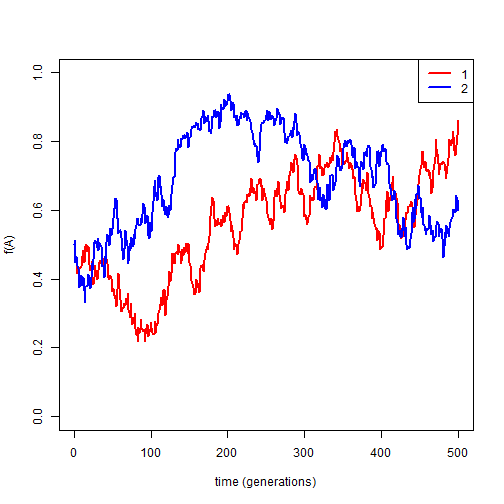``````msd(m=c(0,0),Ne=c(200,200),ngen=500)
``````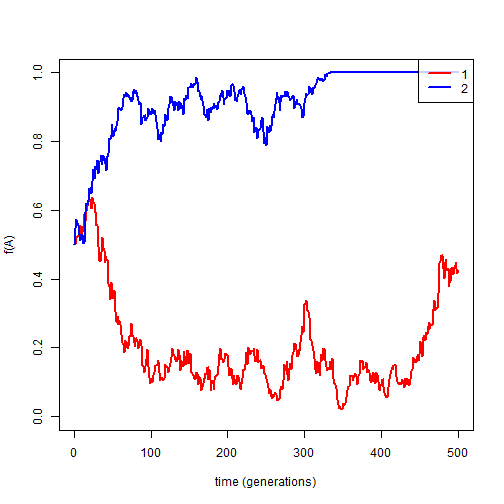Next, migration but no selection:

``````Ne<-c(200,200)
msd(m=1/Ne,Ne=Ne,ngen=500) ## relatively low migration
``````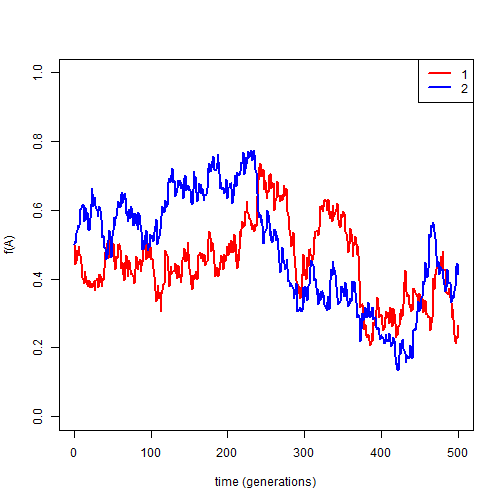``````msd(m=10/Ne,Ne=Ne,ngen=500) ## relatively high migration
``````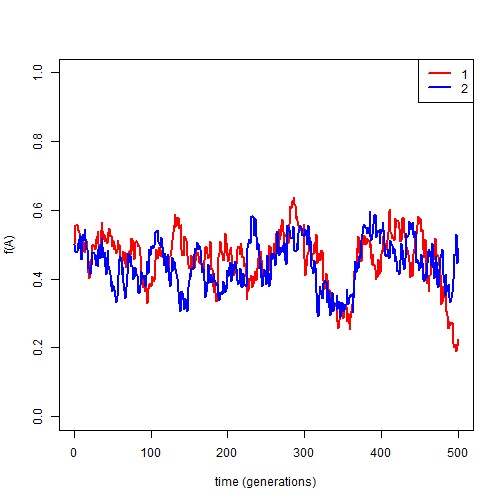Now we're ready to introduce divergent natural selection between populations.

First, for reference, absent migration:

``````msd(p0=c(0.25,0.75),Ne=Ne,w=list(c(1,0.95,0.9),c(0.9,0.95,1)),
m=c(0,0),ngen=500)
``````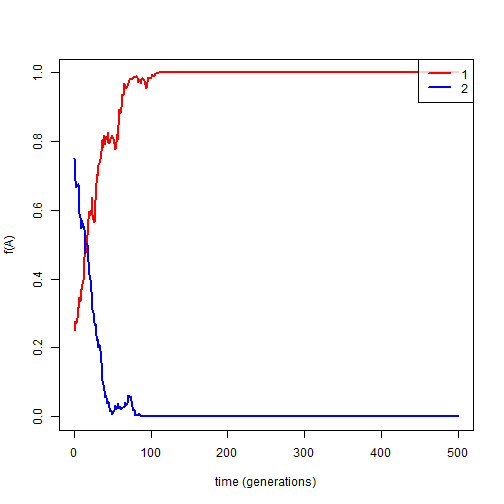Next, with some or a lot of migration:

``````msd(p0=c(0.25,0.75),Ne=Ne,w=list(c(1,0.95,0.9),c(0.9,0.95,1)),
m=1/Ne,ngen=500) ## some migration
``````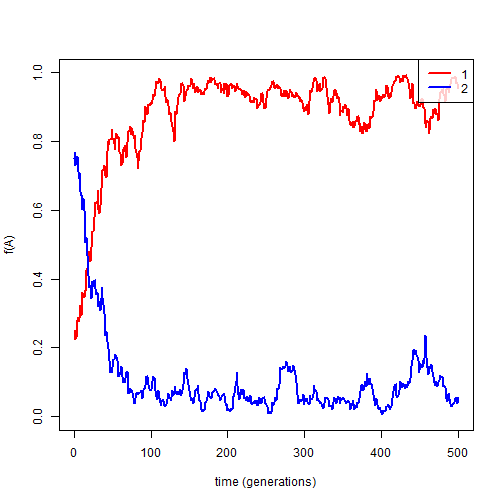``````msd(p0=c(0.25,0.75),Ne=Ne,w=list(c(1,0.95,0.9),c(0.9,0.95,1)),
m=10/Ne,ngen=500) ## lots of migration
``````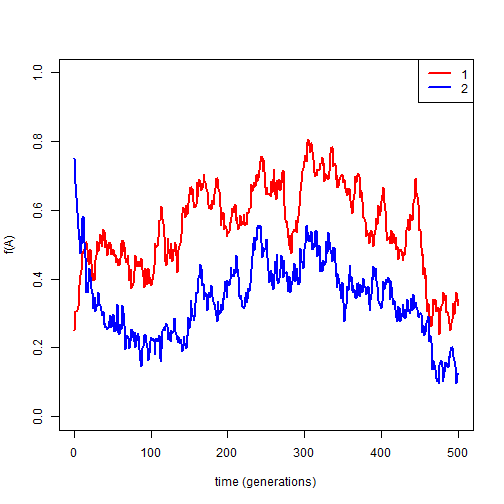Finally, what happens when one population is much larger than the other?

``````msd(p0=c(0.25,0.75),Ne=c(100,1000),w=list(c(1,0.95,0.9),c(0.9,0.95,1)),
m=1/500,ngen=500,show.legend=F)
legend(x="topright",legend=c(expression(paste(N[e],"= 100")),
expression(paste(N[e],"= 1,000"))),lty=1,col=c("red","blue"),
lwd=2,bg=phytools::make.transparent("white", 0.8))
``````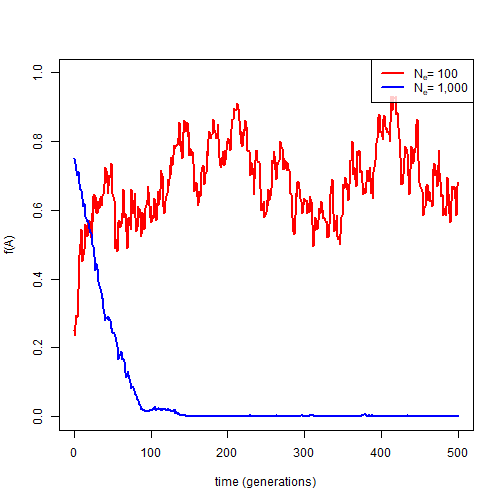Cool.